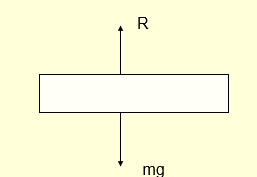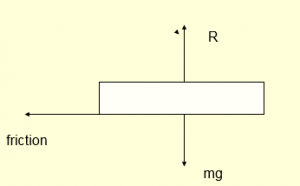Home » Physics » Different type of Forces and their origin

# Different type of Forces and their origin

## Different type of Force and their origin

A) Normal Force: When we put a book on a table ,the molecules of the book exert downward forces on the molecules of the table.The molecules composing the upper layer of the tabletop move downward until the repulsion of the molecules below balances the forces applied by the book.This compression always occur but it is too slight to notice
The following things are good to notice about normal force
a) For a body resting on a surface,Normal force is equal and opposite to the resultant of all the other forces which acts on the body in the direction perpendicular to surface
b) Weight and Normal force are not action reaction pair as they act on same body whereas action -reaction acts on different bodyB) Tension: Tension usually comes under picture for string and rope. The string can be think of small short sections interacting by contact forces.Each section pulls the section to either side of it and by Newton third law,it is pulled by the adjacent section. The magnitude of the forces acting between the section is called Tension. The Following things are good to notice about tension
a) If the tension is uniform,the net string force on each small section is zero and section remains at rest
b) if the string is accelerating ,the tension generally varies along the string
c) If there is external force on the string then also tension varies along the string.

C) Friction Force: Friction force generally comes into picture when one body moves or tries to move along the surface of the second body. Friction always opposes the motion which would occur in its absence. Friction occurs because of inter atomic forces at the actual area of contact on atomic scale.
a) When we try to push a box on the table, if we push the box gently ,the box remains at rest. Force of friction assumes a value equal to the force applied by us. The force of friction cannot increase indefinitely. If we push the box hard,the box start to slideD) Viscous Forces:A body moving through liquid or gas is retarted by the force of viscosity exerted on it by fluid. It arises because a body moving through the medium exerts forces which set the nearby fluid into motion.By newton third law,the fluid exerts a reaction force on the body.
The Following things are good to notice about viscosity
a)Viscous forces are proportional to velocity
b) It always retard the motion

### 1 thought on “Different type of Forces and their origin”

1. Tell me types of force which register in the book.

This site uses Akismet to reduce spam. Learn how your comment data is processed.